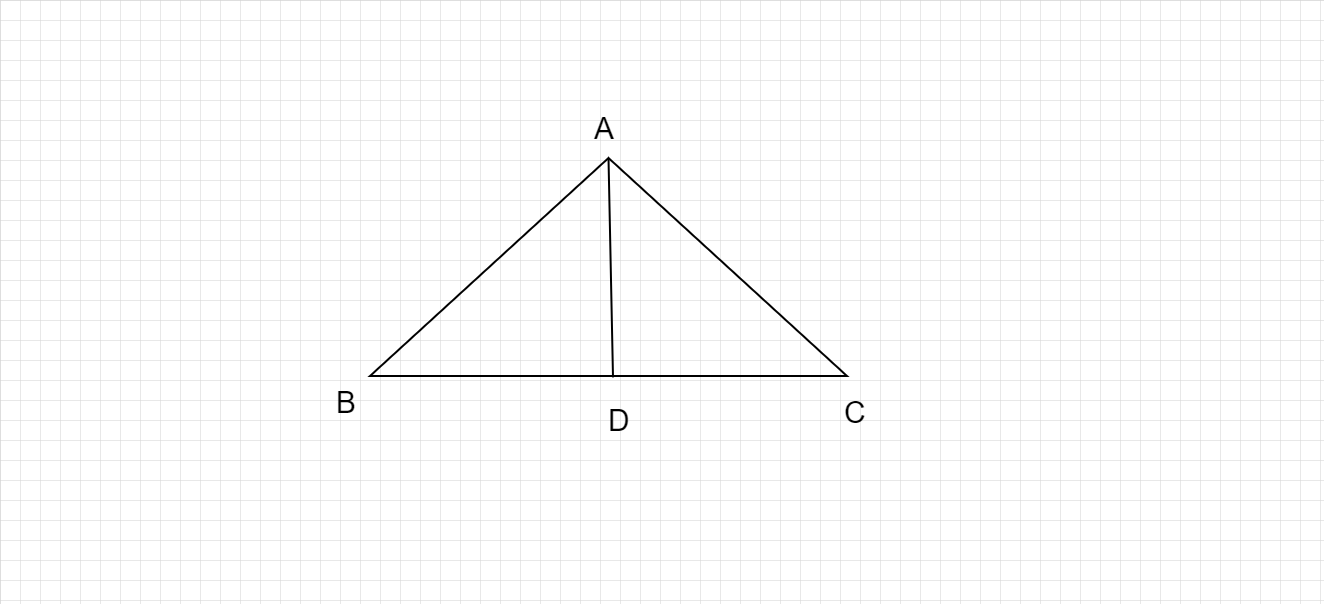"
">

# Count the line segments from the following figure and name them."

Given :

The figure of triangle is given.

To do :

We have to count the line segments from the following figure and name them.

Solution :

In the given figure,

$AB,AC,AD,BC,DC$ and $BD$ are the line segments.

Therefore, the number of line segments in the given figure are $6$.

Updated on: 10-Oct-2022

20 Views